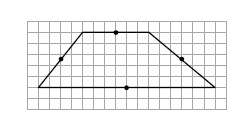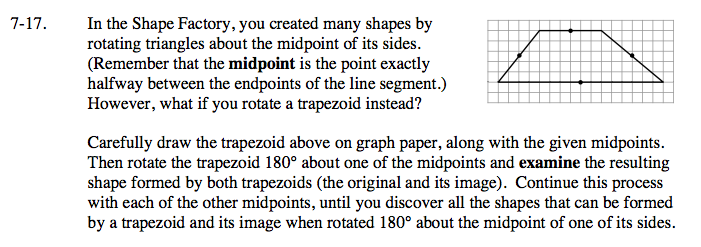### Home > GC > Chapter 7 > Lesson 7.1.2 > Problem7-17

7-17.In the Shape Factory, you created many shapes by rotating triangles about the midpoint of its sides. (Remember that the midpoint is the point exactly halfway between the endpoints of the line segment.) However, what if you rotate a trapezoid instead? 7-17 HW eTool (Desmos). Homework Help ✎

Carefully draw the trapezoid above on graph paper, along with the given midpoints. Then rotate the trapezoid 180° about one of the midpoints and examine the resulting shape formed by both trapezoids (the original and its image). Continue this process with each of the other midpoints, until you discover all the shapes that can be formed by a trapezoid and its image when rotated 180° about the midpoint of one of its sides.Rotating about the midpoint of the base forms a hexagon (one convex and one non-convex).
Rotating the trapezoid about the midpoint of either of the non-base sides forms a parallelogram.

Use the eTool below to rotate the figure and test your answer.
Click the link at right for the full version of the eTool: GC 7-17 HW eTool